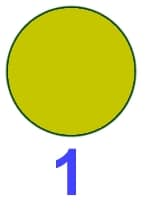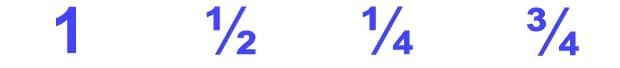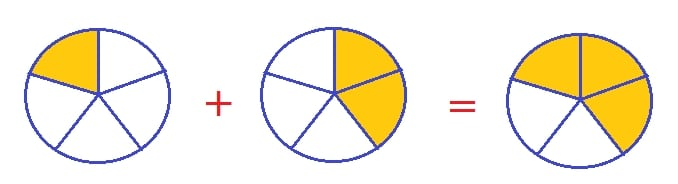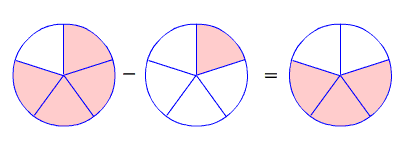# Addition and Subtraction of Fractions of the Same Denominations Primary 3 (Basic 3)

Last Updated on January 25, 2021 by Alabi M. S.

### MATHEMATICS

SECOND TERM

WEEK 2

PRIMARY 3

THEME – BASIC OPERATIONS

PREVIOUS LESSON – Second Term Scheme of Work and Plan Lesson Notes for MATHEMATICS Week 1 to Week 12 Primary Schools

### LEARNING AREA

1. Introductory Activities

2. Addition and Subtraction of Numbers not More Two Digits

3. Introduction to Fractions

4. Addition and Subtraction of Numbers of Fraction

5. Lesson Evaluation and Weekly Assessment (Test)

### PERFORMANCE OBJECTIVES

By the end of the lesson, most should have attained the following objectives (cognitive, affective and psychomotor) and should be able to add and subtract fractions with the same denominator.

### ENTRY BEHAVIOUR

The pupils can add and subtract numbers.

### INSTRUCTIONAL MATERIALS

The teacher will teach the lesson with the aid of Charts and flash cards, etc. Addition cards, Fraction card, Fraction board, Counters, charts, Abacus, etc.

### METHOD OF TEACHING

Choose a suitable and appropriate methods for the lessons.

Note – Irrespective of choosing methods of teaching, always introduce an activities that will arouse pupil’s interest or lead them to the lessons.

### REFERENCE MATERIALS

1. Scheme of Work

2. 9 – Years Basic Education Curriculum

3. Course Book

4. All Relevant Material

5. Online Information – Kids Maths

### LESSON 1 – INTRODUCTORY ACTIVITIES

Teacher’s Introductory Questions – Ask the pupils to solve the following basic addition of numbers.

1. 11 + 9 =

2. 2 + 8 =

3. 4 + 9 =

4. 1 + 8 =

5. 14 + 6 =

Pupil’s Activities – 1. 20, 2. 10, 3. 13, 4. 9 and 5. 20.

Teacher’s activities – Check pupil’s activities and make correction.

Subtraction of Numbers

Teacher’s Introductory Questions – Ask the pupils to solve the following basic subtraction of numbers.

1. 12 – 6 =

2. 15 – 9 =

3. 9 – 5 =

4. 20 – 11 =

5. 13 – 13 =

Pupil’s Activities – 1. 6, 2. 6, 3. 4, 4. 9 and 5. 0.

Teacher’s Activities – Check pupil’s activities and make correction.

Quick Evaluation/Assignment

Complete the following –

1. 11 + (___) = 16

2. (___) – 6 = 14

3. 11 + 11 = (___)

4. (___) + (___) = 15

5. 19 – (___) = 15

Note – Mark and correct on or before the next lesson.

### LESSON 2 – TYPES OF FRACTION

Teacher’s/Pupil’s Activities – Get an object like bread or orange to demonstrate and introduce the concept of fractions.

Also, you can make use of cardboard circle and lead pupils to identify as a whole.

A Whole – One CirclePupil’s Activities – Guide the pupils to identify Fraction as Part of a Whole.

Half of Circle – 1/2Quarter of A Circle – 1/3Three Quarter of A Circle – 3/4Teacher’s remark – When a circle is divided into a number of parts, the fraction shows how many of those parts you have. Therefore, A fraction represents part of a whole.

Teacher’s/Pupil’s Activities – Guide the pupils to identify numerator and denominationTeacher’s remark – fraction has two main parts – numerator and denomination. Fractions are written with the numerator over the denominator and a line in between them and read as 1 over 2, 1 over 4 and 3 over 4.

Teacher’s/Pupil’s Activities – Display a chart showing two (2) bold fractions and ask the pupils to pronounce, examine and notice the similarity and different.

## 3/4 and 4/3

3/4 – 3 over 4

4/3 – 4 over 3

Pupil’s respond – Numerator of 3/4 is less than the numerator of 4/3 while denomination of 3/4 is greater than the denominator of 4/3.

## 7/5 and 5/7

Teacher’s remark – When the numerator is less than the denominator is called PROPER FRACTION. Proper fraction is always less than one. When the numerator is greater than the denominator is called IMPROPER FRACTION. Improper fraction is always greater than one.

### MIXED FRACTIONS

Teacher’s/Pupil’s Activities – Use objects or other materials to demonstrate and guide pupils to identify and pronounce mixed fractions.## 3 whole number, 3 over 4

Teacher’s remark – A mixed fraction had both a whole number part and a fractional part.

QUICK EVALUATION/ASSIGNMENT

Group the following fractions into proper, improper and mixed fractions.

## 2/3, 7/3, 1 2/5, 2 1/2, 7 3/7, 3/7 and 6/5.

1. Proper Fractions –

2. Improper Fractions –

3. Mixed Fractions –

### LESSON 3 – ADDITION AND SUBTRACTION OF FRACTIONS WITH THE SAME DENOMINATIONS

Teacher’s/Pupil’s Activities – Guide the pupils to add the following fractions.1/5 + 2/5

= (1 + 2)/5

= 3/5

Note – Guides the pupils to write in fractions and add.

Subtraction of Fractions

Teacher’s/Pupil’s Activities – Guide the pupils to subtraction the following fractions.4/5 – 1/5

= (4 – 1)/5

= 3/5

Note – Guides the pupils to write in fractions and subtract.

QUICK EVALUATION/ASSIGNMENT

Samples

A. 5/8 + 1/8 = (5 + 1)/8 = 6/8

B. 5/7 – 3/7 = (5 – 3)/7 = 2/7

Exercises

1. 5/8 – 1/8 =

2. 3/7 + 2/7 =

3. 4/9 – 2/9 =

4. 5/11 + 3/11 =

### PRESENTATION

To deliver the lesson, the teacher adopts the following steps:

1. To introduce the lesson, the teacher revises the previous lesson. Based on this, he/she asks the pupils some questions;

2. Guides pupils to identify fraction with same denominator from fraction boards.

Pupil’s Activities – Use fraction board to bring out fractions with the same denominator.

3. Guides pupils to add fractions having the same denominator:

3⁄6 + 2⁄6 =

3⁄5 + 2⁄5 =

Pupil’s Activities – Add fraction with the same denominator.

4. Teacher’s/Activities – Quantitative Reasonings

### CONCLUSION

• To conclude the lesson for the week, the teacher revises the entire lesson and links it to the following week’s lesson.

### LESSON EVALUATION

1. add given fractions with the same denominator.

2. identify the total parts to be coloured when a cardboard is divided into 2⁄6 + 3⁄6 = 5⁄6.

error: